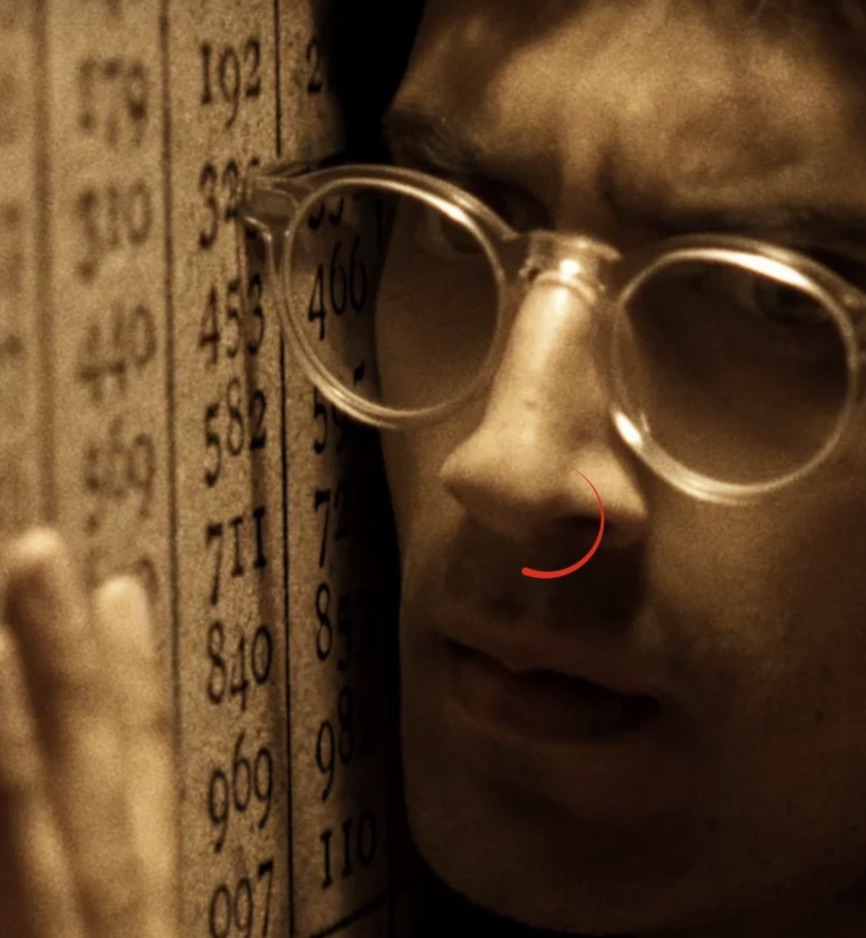# Benford's law image tool

Written by Walter on 26/01/2021​​​​

So this weekend was watching some Netflix. When I stumbled on this show called Connected, I got intreaged during the Digits episode. So benford's law was being presented as being something that connects us all and can do fraud detection and also detect if images are tampered with. When I heard the algorithm as they explained it in lamens terms I thought 1. no way. 2. That's super easy to implement. 3. Ready set go, and then I proceeded to hack together a little benford image analysis tool in a few hours

After I got it working on some random digits. I downloaded a nice nature image from one of my facebook friends, fired up the tool and... OMG it 'kinda' really works ;). Well I must have been extremely lucky or it regularly does actually show a similar curve described Benford's law.

When doing 1 million random digits you get a flat line basically 1..9 are all equally represented. Now when doing the average of the most significand digit in the pixels of an image you'd just expect just similar random things. Like some images showing flat lines, some showing random stuff. But low and behold when running this on a picture taken in nature. It did indeed give back the curve that the tv show predicts. That's quite a coincidence.

However it's not all good news. After editing the image and re-saving + regenerating the benford graph you couldn't really tell the image was tampered with. The curve kept being about the same for the edited image.

Also some images give the nice curve but I was able to find plenty of real images that gave different curves (similar to the benford prediction but nearly not enough to call it totally wicked awesome). Anyway I put the project in my hobby projects and wrote some more about it here:
Benfords law for images and the source code is on github img2benford.

In summary: no it's not something crazy like in that creepy/scary movie called Pi from Clint Mansell but it does indeed have some results you wouldn't expect from such a simple algorithm.

Now go download the git repo, and compile it while you've got the movie song from Pi playing. Fire up ./img2benford and be amased... or disappointed, whatever it gives, it's something geek over or talk about with some nerdy friends ;).

Before I forget, the movie Pi is also worth a watch if you don't know it already... And don't go flipping out when using this tool, like Max did. Yes there are correlations everywhere. "There are lies, big lies and then there are statistics" is one of the one-liners that pops into my mind ;)

Here's that first example I tried on the first nature picture I found on facebook and that worked like a charm (it's included in the git repo in the tests folder):

``````
./img2benford tests/nature_image.jpg

#########
#########
#########
#########
#########   #########
#########   #########
#########   #########
#########   #########
#########   #########
#########   #########
#########   #########
#########   #########
#########   #########   #########
#########   #########   #########
#########   #########   #########
#########   #########   #########   #########
#########   #########   #########   #########
#########   #########   #########   #########   #########
#########   #########   #########   #########   #########
#########   #########   #########   #########   #########
#########   #########   #########   #########   #########   #########
#########   #########   #########   #########   #########   #########   #########
#########   #########   #########   #########   #########   #########   #########   #########   #########
#########   #########   #########   #########   #########   #########   #########   #########   #########
#########   #########   #########   #########   #########   #########   #########   #########   #########
1 (25)      2 (21)      3 (13)      4 (10)      5 (8)       6 (5)       7 (4)       8 (3)       9 (3)

benford count 1 = 648313 percentage = 25
benford count 2 = 531042 percentage = 21
benford count 3 = 351602 percentage = 13
benford count 4 = 273866 percentage = 10
benford count 5 = 211944 percentage = 8
benford count 6 = 127823 percentage = 5
benford count 7 = 106355 percentage = 4
benford count 8 = 89524 percentage = 3
benford count 9 = 91162 percentage = 3
```
```